# RD Sharma Solutions For Class 7 Maths Chapter 15 Properties of Triangles Exercise 15.5

Students can view and download the RD Sharma Solutions for Class 7 Maths Exercise 15.5 of Chapter 15 Properties of Triangles from the links provided below. The syllabus given by the CBSE for all the classes is completely based on the RD Sharma textbook. Hence, exam preparation using the RD Sharma Solutions for Class 7 will definitely provide students with an advantage of scoring well. This exercise explains Pythagoras theorem. Some of the topics of this exercise are listed below.

• Pythagoras theorem
• Corollary based on Pythagoras theorem
• Converse of Pythagoras theorem
• Pythagorean triplets

## Download the PDF of RD Sharma Solutions For Class 7 Maths Chapter 15 – Properties of Triangles Exercise 15.5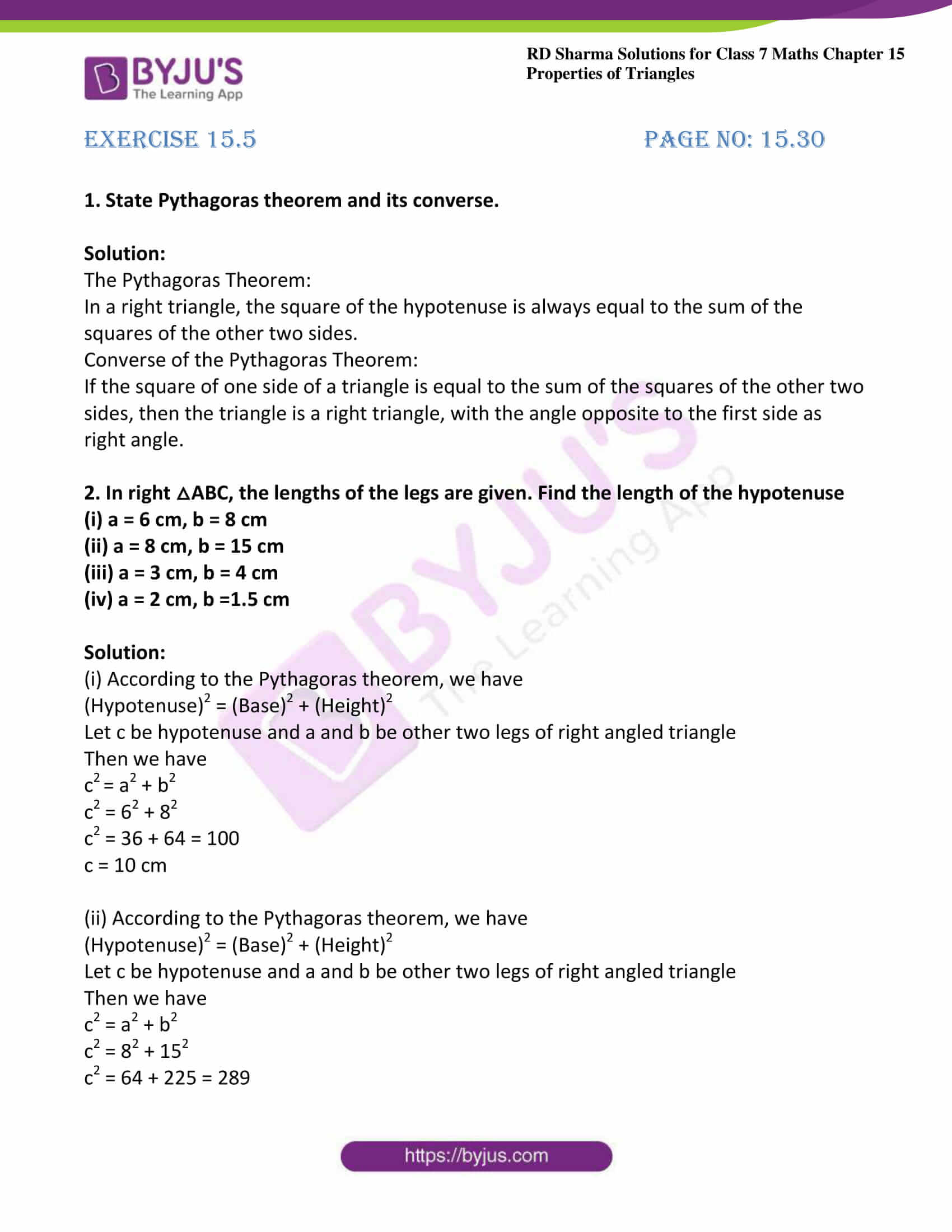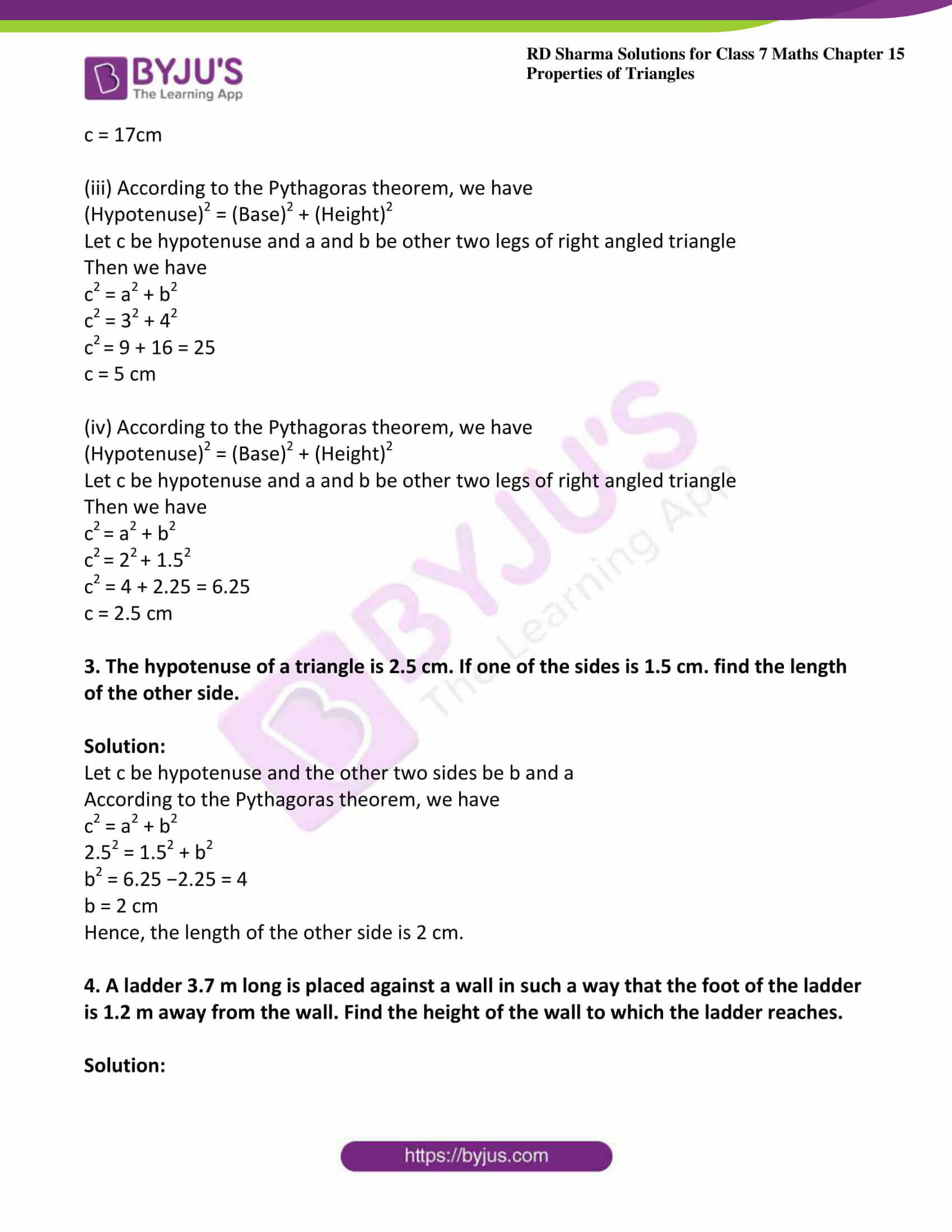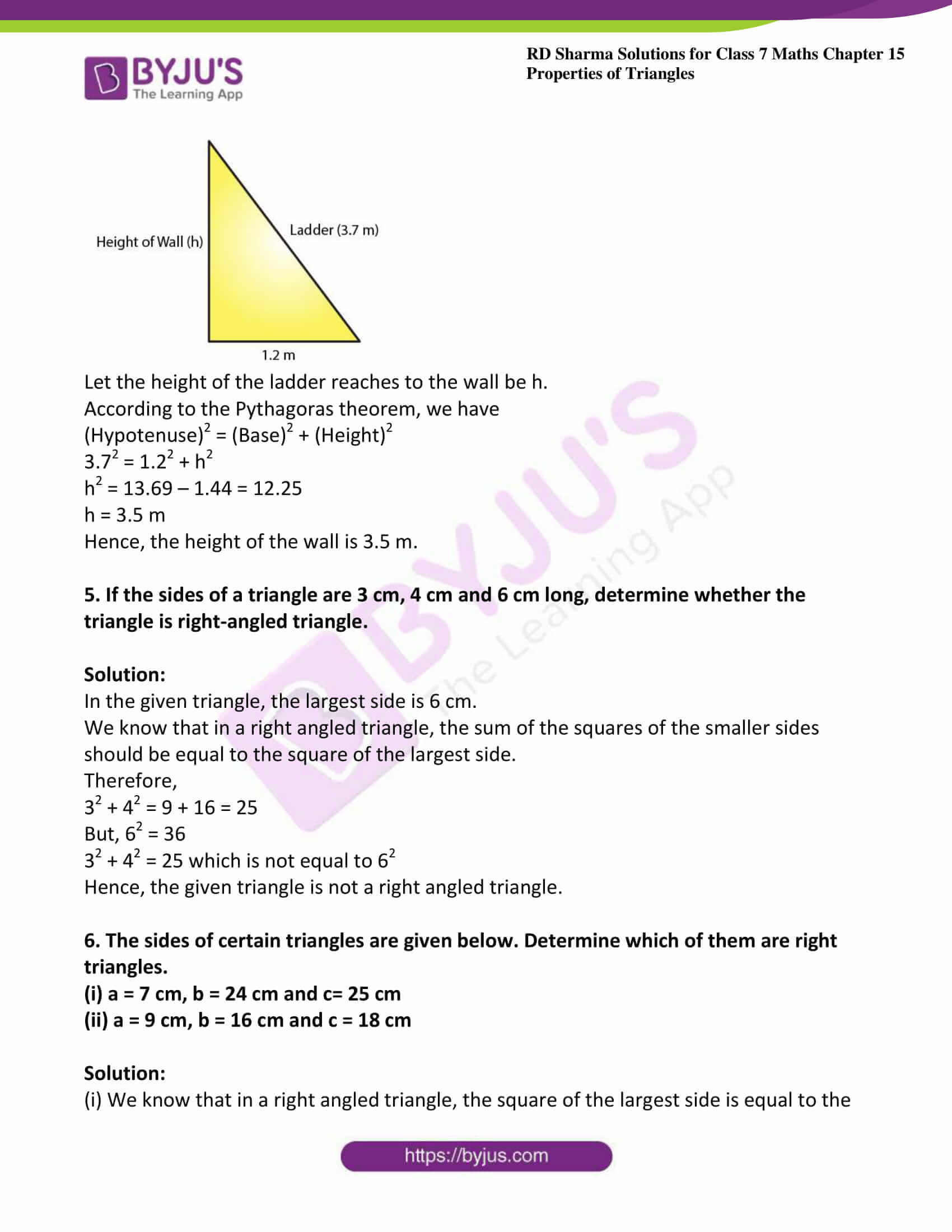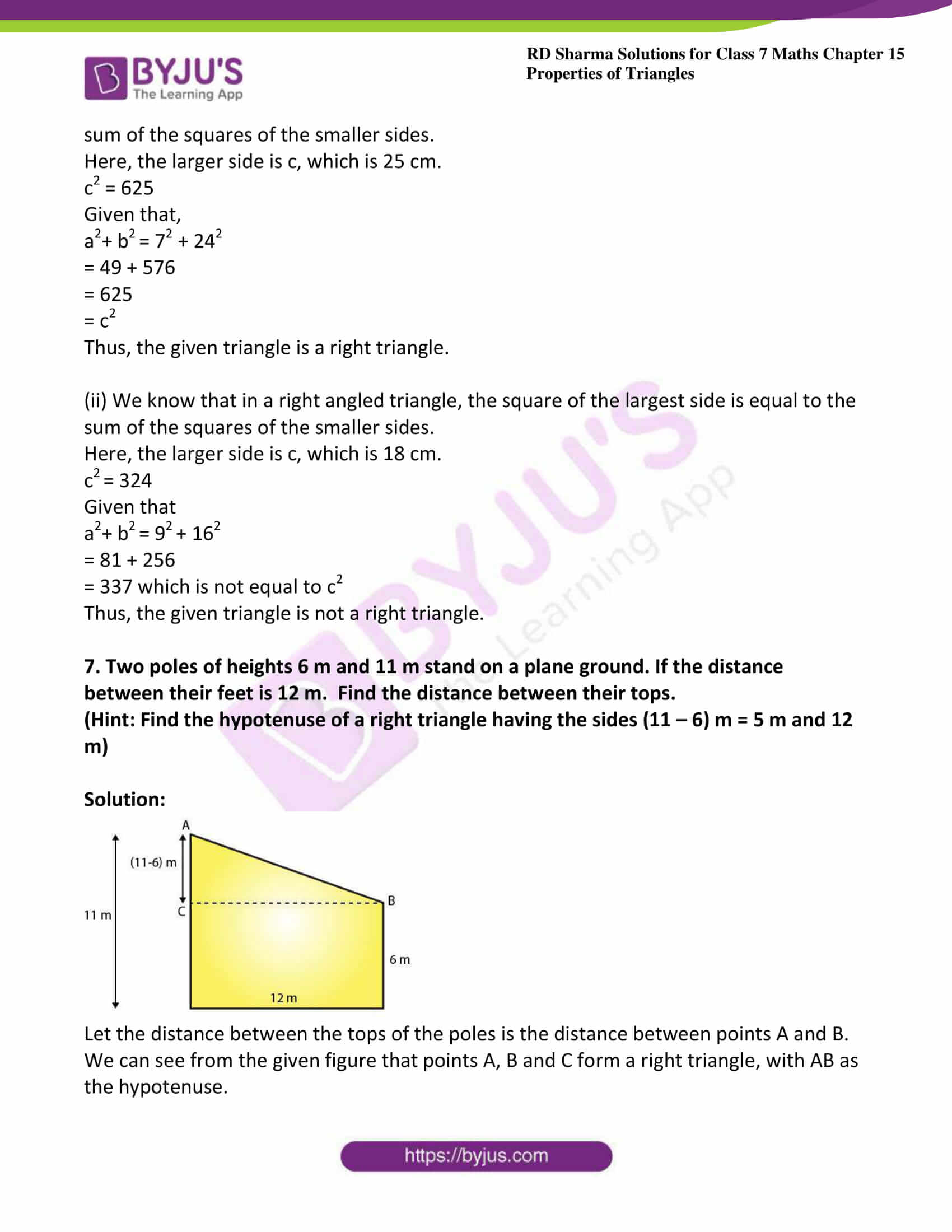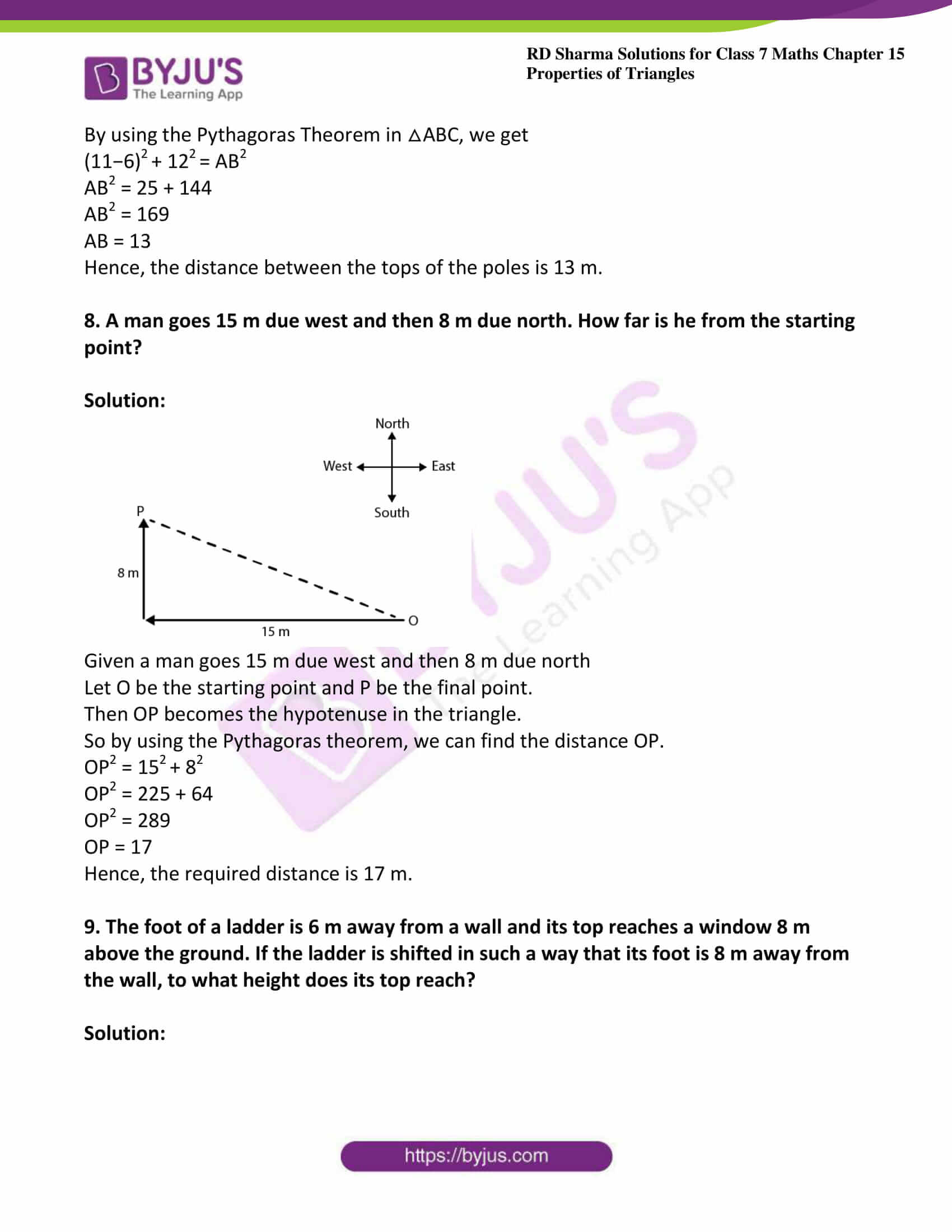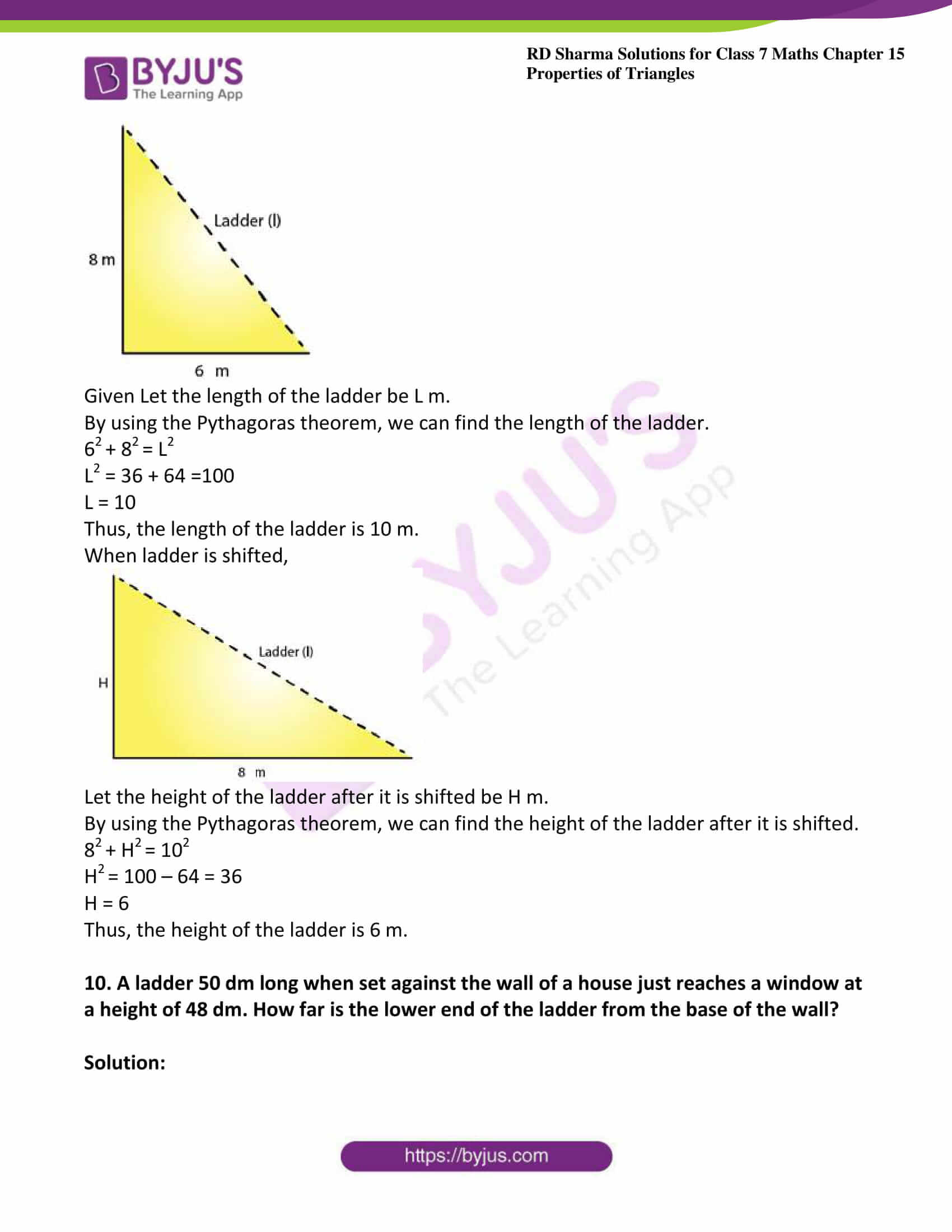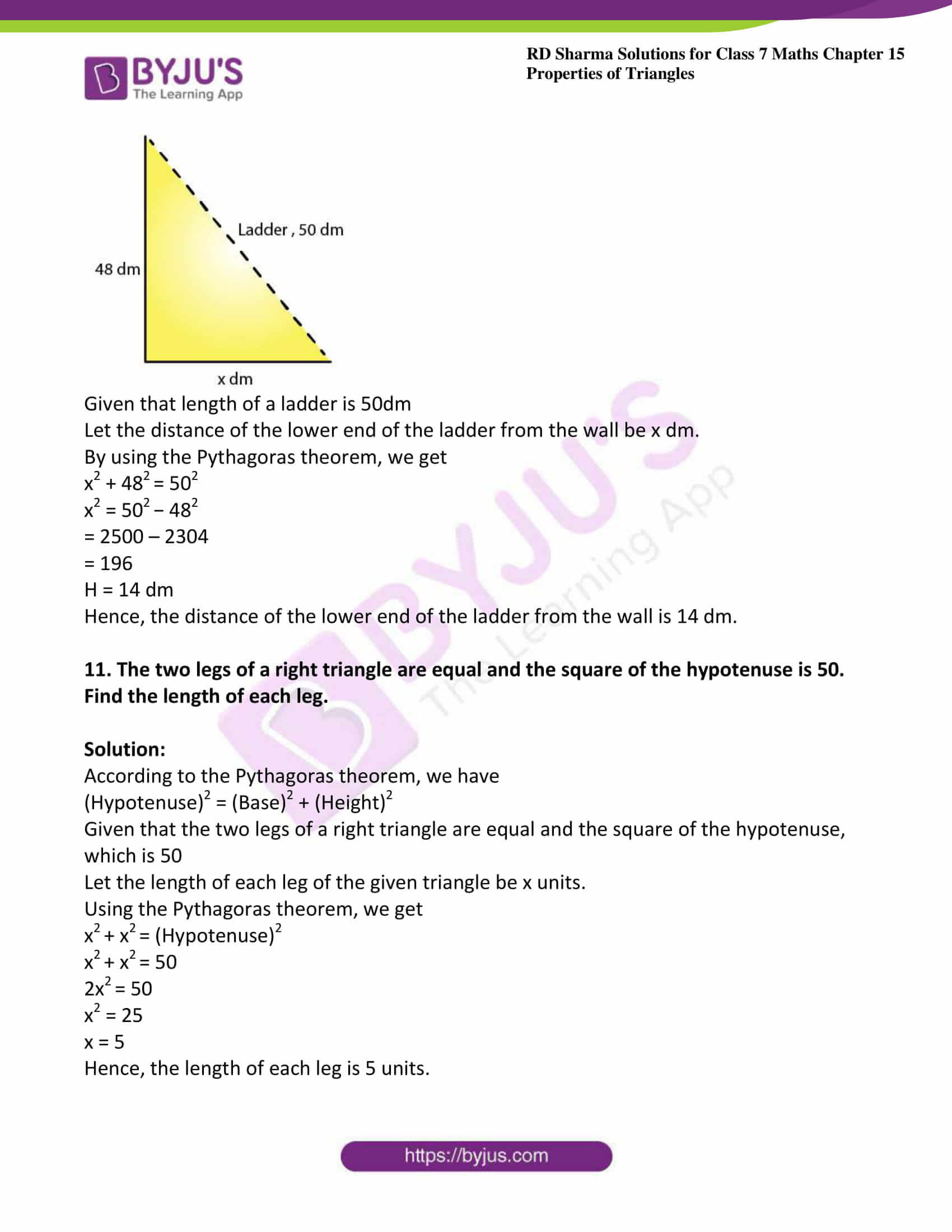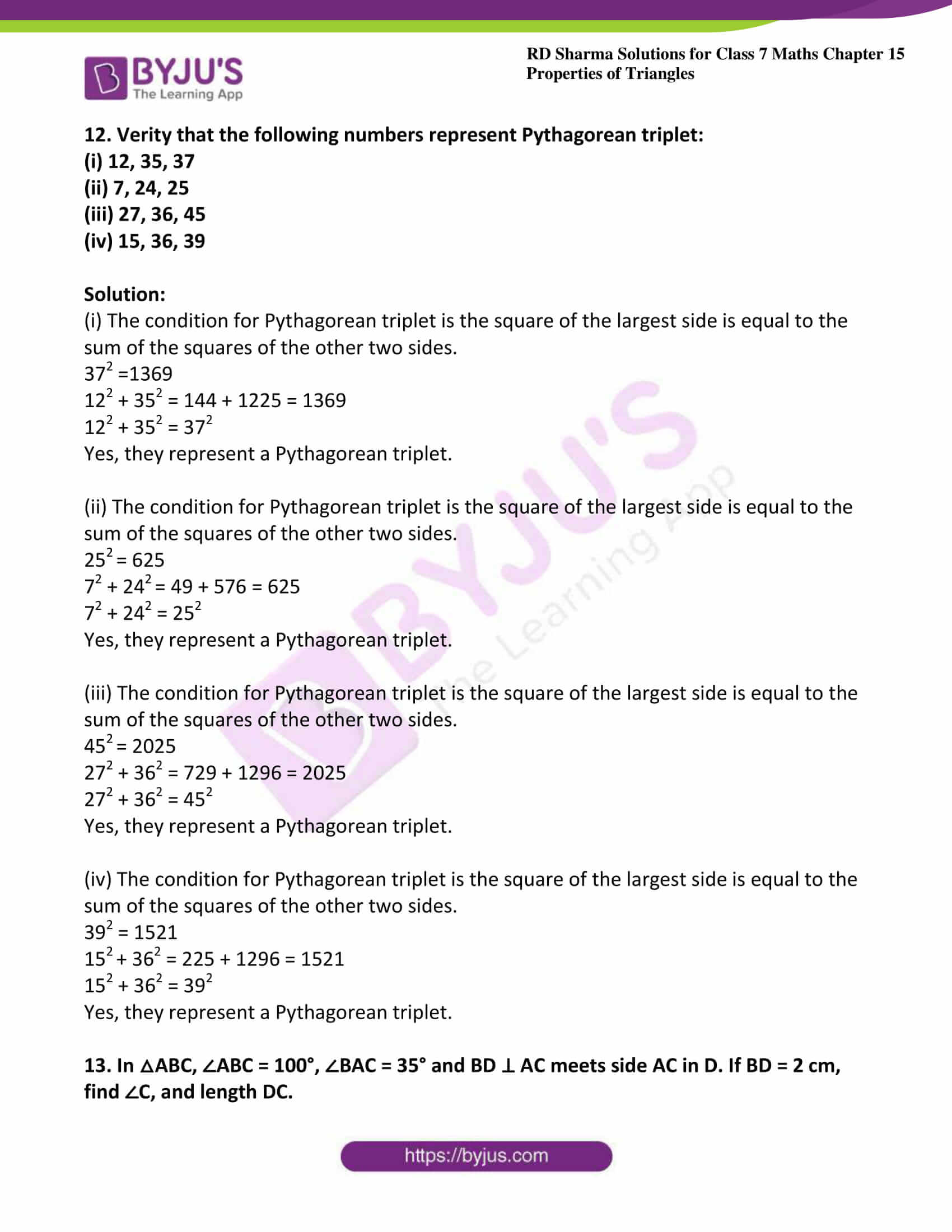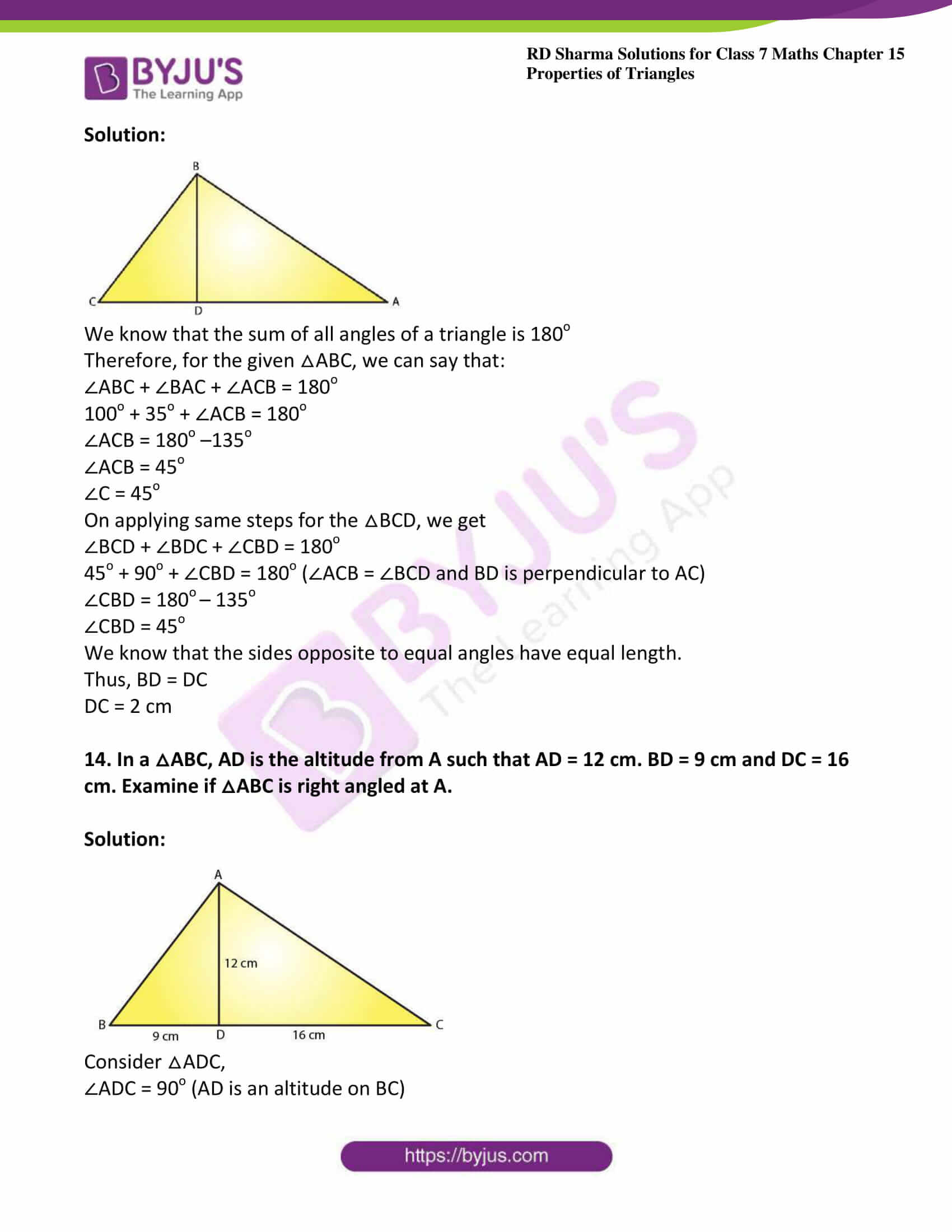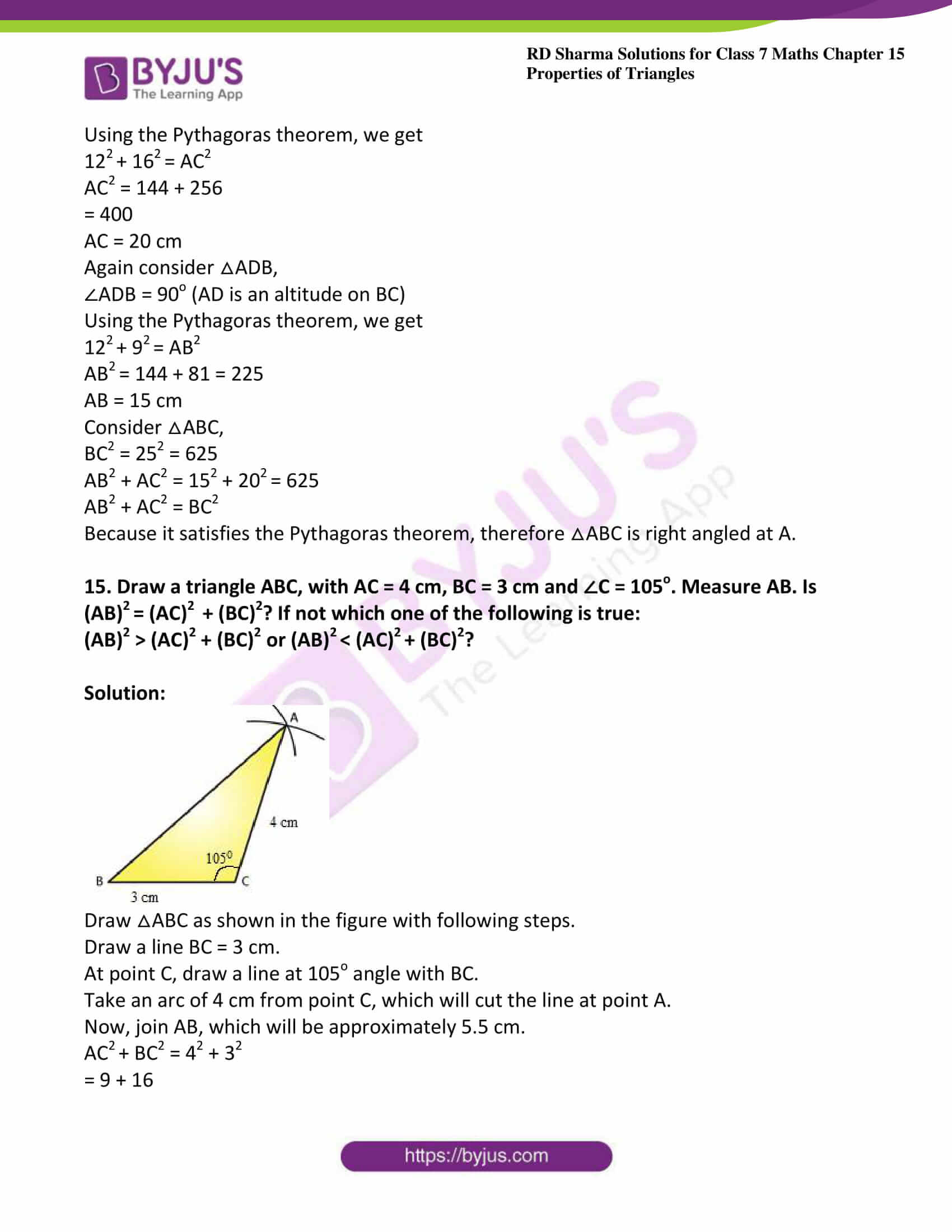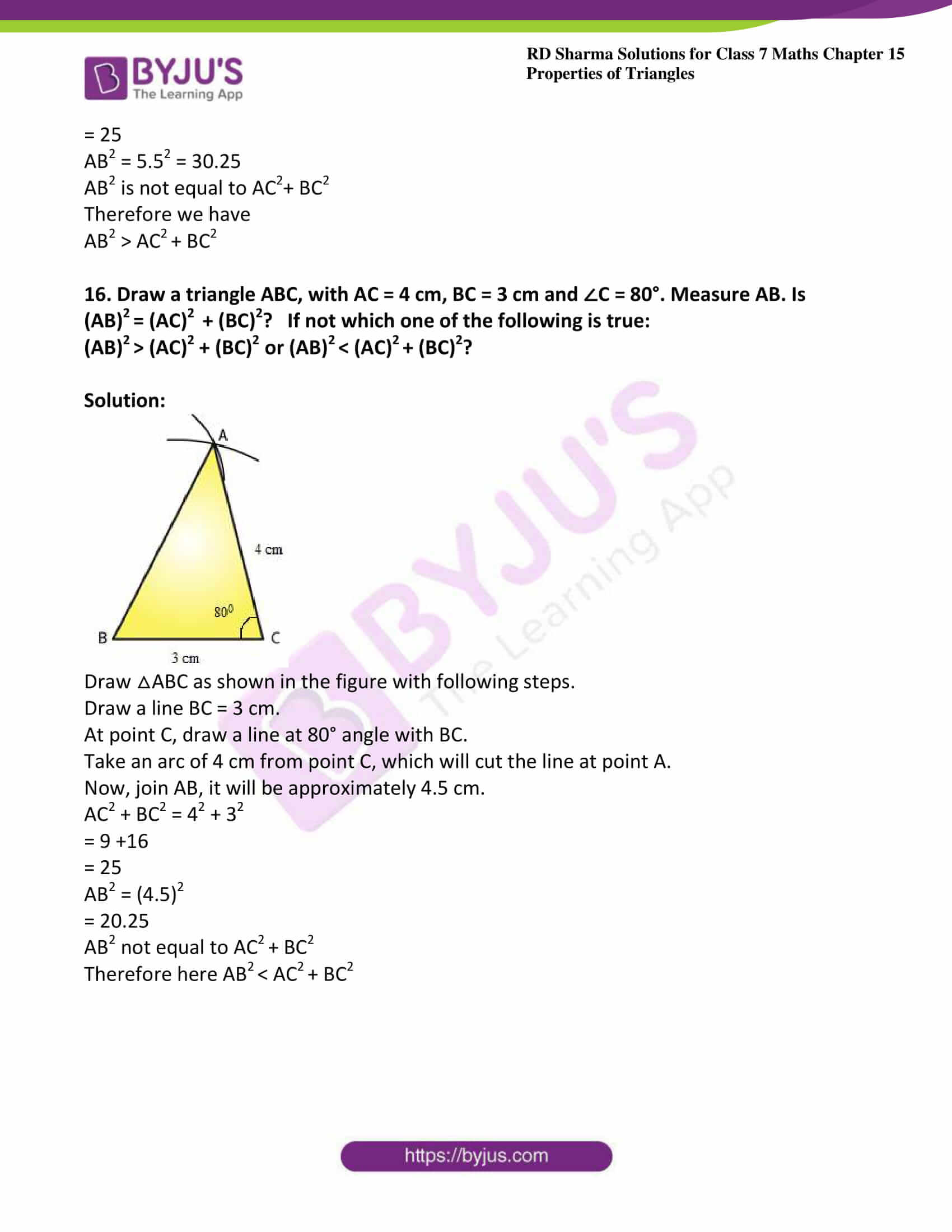### Access answers to Maths RD Sharma Solutions For Class 7 Chapter 15 – Properties of Triangles Exercise 15.5

Exercise 15.5 Page No: 15.30

1. State Pythagoras theorem and its converse.

Solution:

The Pythagoras Theorem:

In a right triangle, the square of the hypotenuse is always equal to the sum of the squares of the other two sides.

Converse of the Pythagoras Theorem:

If the square of one side of a triangle is equal to the sum of the squares of the other two sides, then the triangle is a right triangle, with the angle opposite to the first side as right angle.

2. In right △ABC, the lengths of the legs are given. Find the length of the hypotenuse

(i) a = 6 cm, b = 8 cm

(ii) a = 8 cm, b = 15 cm

(iii) a = 3 cm, b = 4 cm

(iv) a = 2 cm, b =1.5 cm

Solution:

(i) According to the Pythagoras theorem, we have

(Hypotenuse)2 = (Base)2 + (Height)2

Let c be hypotenuse and a and b be other two legs of right angled triangle

Then we have

c= a2 + b2

c2 = 62 + 82

c2 = 36 + 64 = 100

c = 10 cm

(ii) According to the Pythagoras theorem, we have

(Hypotenuse)2 = (Base)2 + (Height)2

Let c be hypotenuse and a and b be other two legs of right angled triangle

Then we have

c2 = a2 + b2

c2 = 82 + 152

c2 = 64 + 225 = 289

c = 17cm

(iii) According to the Pythagoras theorem, we have

(Hypotenuse)2 = (Base)2 + (Height)2

Let c be hypotenuse and a and b be other two legs of right angled triangle

Then we have

c2 = a2 + b2

c2 = 32 + 42

c= 9 + 16 = 25

c = 5 cm

(iv) According to the Pythagoras theorem, we have

(Hypotenuse)2 = (Base)2 + (Height)2

Let c be hypotenuse and a and b be other two legs of right angled triangle

Then we have

c= a2 + b2

c= 2+ 1.52

c2 = 4 + 2.25 = 6.25

c = 2.5 cm

3. The hypotenuse of a triangle is 2.5 cm. If one of the sides is 1.5 cm. find the length of the other side.

Solution:

Let c be hypotenuse and the other two sides be b and a

According to the Pythagoras theorem, we have

c2 = a2 + b2

2.52 = 1.52 + b2

b2 = 6.25 −2.25 = 4

b = 2 cm

Hence, the length of the other side is 2 cm.

4. A ladder 3.7 m long is placed against a wall in such a way that the foot of the ladder is 1.2 m away from the wall. Find the height of the wall to which the ladder reaches.

Solution: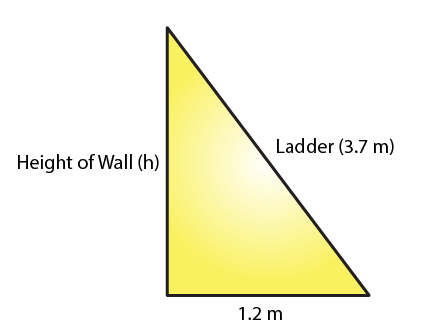Let the height of the ladder reaches to the wall be h.

According to the Pythagoras theorem, we have

(Hypotenuse)2 = (Base)2 + (Height)2

3.72 = 1.22 + h2

h2 = 13.69 – 1.44 = 12.25

h = 3.5 m

Hence, the height of the wall is 3.5 m.

5. If the sides of a triangle are 3 cm, 4 cm and 6 cm long, determine whether the triangle is right-angled triangle.

Solution:

In the given triangle, the largest side is 6 cm.

We know that in a right angled triangle, the sum of the squares of the smaller sides should be equal to the square of the largest side.

Therefore,

32 + 42 = 9 + 16 = 25

But, 62 = 36

32 + 42 = 25 which is not equal to 62

Hence, the given triangle is not a right angled triangle.

6. The sides of certain triangles are given below. Determine which of them are right triangles.

(i) a = 7 cm, b = 24 cm and c= 25 cm

(ii) a = 9 cm, b = 16 cm and c = 18 cm

Solution:

(i) We know that in a right angled triangle, the square of the largest side is equal to the sum of the squares of the smaller sides.

Here, the larger side is c, which is 25 cm.

c2 = 625

Given that,

a2+ b= 72 + 242

= 49 + 576

= 625

= c2

Thus, the given triangle is a right triangle.

(ii) We know that in a right angled triangle, the square of the largest side is equal to the sum of the squares of the smaller sides.

Here, the larger side is c, which is 18 cm.

c= 324

Given that

a2+ b= 92 + 16

= 81 + 256

= 337 which is not equal to c2

Thus, the given triangle is not a right triangle.

7. Two poles of heights 6 m and 11 m stand on a plane ground. If the distance between their feet is 12 m.  Find the distance between their tops.

(Hint: Find the hypotenuse of a right triangle having the sides (11 – 6) m = 5 m and 12 m)

Solution: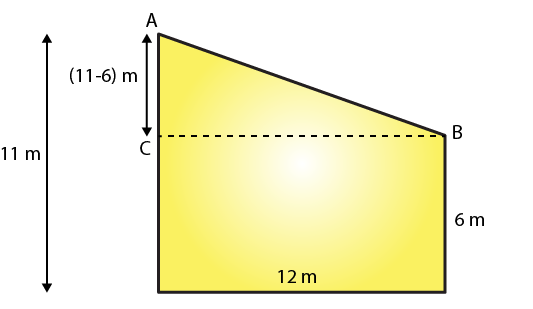Let the distance between the tops of the poles is the distance between points A and B.

We can see from the given figure that points A, B and C form a right triangle, with AB as the hypotenuse.

By using the Pythagoras Theorem in △ABC, we get

(11−6)+ 12= AB2

AB2 = 25 + 144

AB2 = 169

AB = 13

Hence, the distance between the tops of the poles is 13 m.

8. A man goes 15 m due west and then 8 m due north. How far is he from the starting point?

Solution: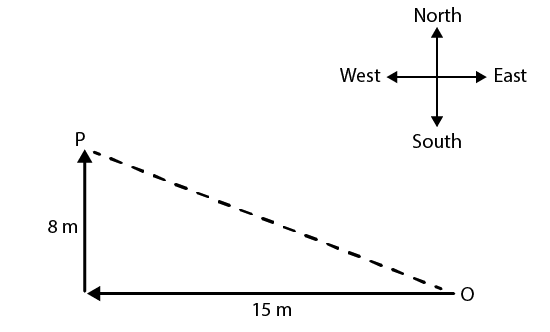Given a man goes 15 m due west and then 8 m due north

Let O be the starting point and P be the final point.

Then OP becomes the hypotenuse in the triangle.

So by using the Pythagoras theorem, we can find the distance OP.

OP2 = 15+ 82

OP2 = 225 + 64

OP2 = 289

OP = 17

Hence, the required distance is 17 m.

9. The foot of a ladder is 6 m away from a wall and its top reaches a window 8 m above the ground. If the ladder is shifted in such a way that its foot is 8 m away from the wall, to what height does its top reach?

Solution: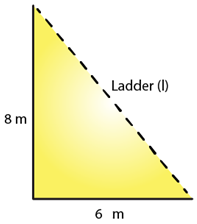Given Let the length of the ladder be L m.

By using the Pythagoras theorem, we can find the length of the ladder.

6+ 8= L2

L2 = 36 + 64 =100

L = 10

Thus, the length of the ladder is 10 m.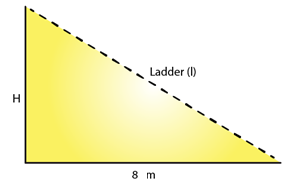Let the height of the ladder after it is shifted be H m.

By using the Pythagoras theorem, we can find the height of the ladder after it is shifted.

8+ H= 102

H= 100 – 64 = 36

H = 6

Thus, the height of the ladder is 6 m.

10. A ladder 50 dm long when set against the wall of a house just reaches a window at a height of 48 dm. How far is the lower end of the ladder from the base of the wall?

Solution: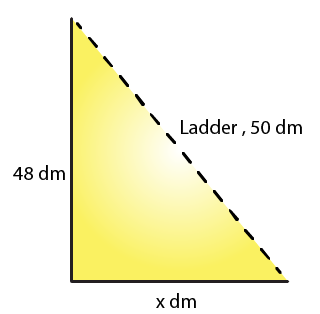Given that length of a ladder is 50dm

Let the distance of the lower end of the ladder from the wall be x dm.

By using the Pythagoras theorem, we get

x2 + 48= 502

x2 = 50− 48

= 2500 – 2304

= 196

H = 14 dm

Hence, the distance of the lower end of the ladder from the wall is 14 dm.

11. The two legs of a right triangle are equal and the square of the hypotenuse is 50. Find the length of each leg.

Solution:

According to the Pythagoras theorem, we have

(Hypotenuse)2 = (Base)2 + (Height)2

Given that the two legs of a right triangle are equal and the square of the hypotenuse, which is 50

Let the length of each leg of the given triangle be x units.

Using the Pythagoras theorem, we get

x+ x= (Hypotenuse)2

x+ x= 50

2x= 50

x2 = 25

x = 5

Hence, the length of each leg is 5 units.

12. Verity that the following numbers represent Pythagorean triplet:

(i) 12, 35, 37

(ii) 7, 24, 25

(iii) 27, 36, 45

(iv) 15, 36, 39

Solution:

(i) The condition for Pythagorean triplet is the square of the largest side is equal to the sum of the squares of the other two sides.

372 =1369

122 + 352 = 144 + 1225 = 1369

122 + 352 = 372

Yes, they represent a Pythagorean triplet.

(ii) The condition for Pythagorean triplet is the square of the largest side is equal to the sum of the squares of the other two sides.

25= 625

72 + 24= 49 + 576 = 625

72 + 242 = 252

Yes, they represent a Pythagorean triplet.

(iii) The condition for Pythagorean triplet is the square of the largest side is equal to the sum of the squares of the other two sides.

45= 2025

272 + 362 = 729 + 1296 = 2025

272 + 362 = 452

Yes, they represent a Pythagorean triplet.

(iv) The condition for Pythagorean triplet is the square of the largest side is equal to the sum of the squares of the other two sides.

392 = 1521

15+ 362 = 225 + 1296 = 1521

152 + 362 = 392

Yes, they represent a Pythagorean triplet.

13. In △ABC, ∠ABC = 100°, ∠BAC = 35° and BD ⊥ AC meets side AC in D. If BD = 2 cm, find ∠C, and length DC.

Solution: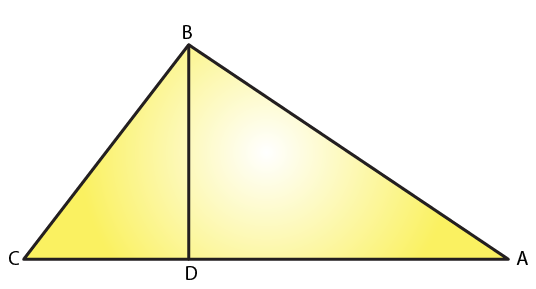We know that the sum of all angles of a triangle is 180o

Therefore, for the given △ABC, we can say that:

∠ABC + ∠BAC + ∠ACB = 180o

100o + 35o + ∠ACB = 180o

∠ACB = 180o –135o

∠ACB = 45o

∠C = 45o

On applying same steps for the △BCD, we get

∠BCD + ∠BDC + ∠CBD = 180o

45o + 90o + ∠CBD = 180o (∠ACB = ∠BCD and BD is perpendicular to AC)

∠CBD = 180o – 135o

∠CBD = 45o

We know that the sides opposite to equal angles have equal length.

Thus, BD = DC

DC = 2 cm

14. In a △ABC, AD is the altitude from A such that AD = 12 cm. BD = 9 cm and DC = 16 cm. Examine if △ABC is right angled at A.

Solution: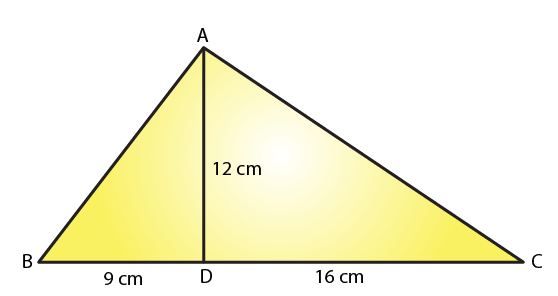Using the Pythagoras theorem, we get

12+ 16= AC2

AC2 = 144 + 256

= 400

AC = 20 cm

Using the Pythagoras theorem, we get

12+ 9= AB2

AB= 144 + 81 = 225

AB = 15 cm

Consider △ABC,

BC2 = 252 = 625

AB2 + AC2 = 152 + 20= 625

AB2 + AC2 = BC2

Because it satisfies the Pythagoras theorem, therefore △ABC is right angled at A.

15. Draw a triangle ABC, with AC = 4 cm, BC = 3 cm and ∠C = 105o. Measure AB. Is (AB)= (AC) + (BC)2? If not which one of the following is true:

(AB)2 > (AC)2 + (BC)2 or (AB)< (AC)+ (BC)2?

Solution: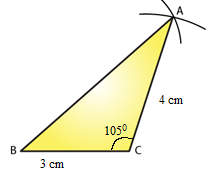Draw △ABC as shown in the figure with following steps.

Draw a line BC = 3 cm.

At point C, draw a line at 105o angle with BC.

Take an arc of 4 cm from point C, which will cut the line at point A.

Now, join AB, which will be approximately 5.5 cm.

AC+ BC2 = 42 + 3

= 9 + 16

= 25

AB2 = 5.52 = 30.25

AB2 is not equal to AC2+ BC2

Therefore we have

AB2 > AC+ BC2

16. Draw a triangle ABC, with AC = 4 cm, BC = 3 cm and ∠C = 80°. Measure AB. Is (AB)= (AC) + (BC)2? If not which one of the following is true:

(AB)> (AC)2 + (BC)2 or (AB)< (AC)+ (BC)2?

Solution: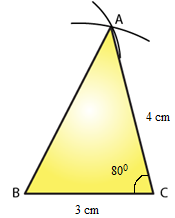Draw △ABC as shown in the figure with following steps.

Draw a line BC = 3 cm.

At point C, draw a line at 80° angle with BC.

Take an arc of 4 cm from point C, which will cut the line at point A.

Now, join AB, it will be approximately 4.5 cm.

AC2 + BC2 = 42 + 32

= 9 +16

= 25

AB2 = (4.5)2

= 20.25

AB2 not equal to AC+ BC2

Therefore here AB< AC+ BC2